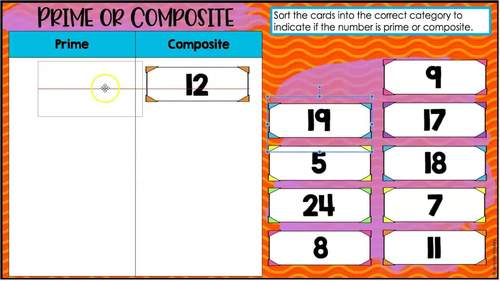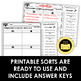# 4th Grade Math Sorts - Digital Math Sorts Included for Distance LearningSubject
Resource Type
File Type
PDF (19 MB|36 Math Sorts + Digital Versions)
Standards
\$6.00
Also included in:
1. Do you want your math stations and guided math centers done for the year? This 4th grade guided math bundle is the only resource you will need to have your math centers done for the year!NOTE: I have added a GROWING set of Digital Math Google Forms to this resource. The price will increase to \$95 by
\$160.50
\$86.00
Save \$74.50
• Product Description
• Standards

Math sorts are an excellent way to push your students' learning and thinking as they explore new math topics. This print and digital math resource includes 4th grade math sorts aligned to the common core standards (but can be used with other standards as well).

Need 3rd or 5th Grade Math Sorts?

• This resource includes 36 math sorts, one for each standard/substandard for 4th grade common core.

• The math sorts are included in printable format and a digital format for use with Google Slides.

• Answer keys for the math sorts are included in the PDF file.

Each printable math sort is 2 pages: 1 page for the pockets and directions and one page for the sorting cards.

Using the Printable Math Sorts

These sorts work perfectly in an interactive notebook, but would also work in a center. You could even glue the pockets to a file folder and create an instant sorting game for fun review. I have included a few generic recording sheets if you wish to go with this option.

Printing Tip: Print the sorts with four pockets at 70% and the sorts with three pockets at 80% to fit all the pockets on one page in a spiral notebook.

The digital math sorts are included for use with Google Slides.

The math sorts are all separated by skill. The links to access the digital math sorts can be found on pages 3 and 4.

4th Grade Math Skills (One Sort Per Skill):

• Multiplicative Comparison Statements
• One Step vs Multi-Step Problems
• Working with Remainders
• Factor Pairs
• Prime or Composite
• Patterns
• Understanding Place Value
• Representing Whole Numbers
• Comparing Whole Numbers
• Rounding Whole Numbers
• Adding and Subtracting Whole Numbers
• Multiplying Whole Numbers
• Dividing Whole Numbers
• Equivalent Fractions
• More Equivalent Fractions
• Comparing Fractions
• Decomposing Fractions
• Adding and Subtracting Mixed Numbers
• Fraction Word Problems
• Multiplying with Fractions
• Working with Fractions with Denominators of 10 and 100
• Fractions and Decimals
• Comparing Decimals
• Comparing Measurement Conversions
• Measurement Word Problems
• Area and Perimeter
• Line Plots (Printable Only)
• Rays and Angles
• Types of Angles
• Types of Triangles
• Types of Lines
• Lines in 2-D Shapes
• Symmetry

Using the 4th Grade Math Sorts

The math sorts could be used as part of your interactive math lesson, for RTI or reteaching, in math centers, tutoring, or even homework.

The students could create a notebook of sorts that they continually practiced and sorted for homework throughout the year.

The digital math sorts can be used as a digital math center, digital homework option, or for distance learning.

Make sure you grab the FREE Launching Math Centers Starter Pack with review centers to help you launch your centers. You can grab it by clicking here.

Compare two decimals to hundredths by reasoning about their size. Recognize that comparisons are valid only when the two decimals refer to the same whole. Record the results of comparisons with the symbols >, =, or <, and justify the conclusions, e.g., by using a visual model.
Use decimal notation for fractions with denominators 10 or 100. For example, rewrite 0.62 as 62/100; describe a length as 0.62 meters; locate 0.62 on a number line diagram.
Express a fraction with denominator 10 as an equivalent fraction with denominator 100, and use this technique to add two fractions with respective denominators 10 and 100. For example, express 3/10 as 30/100, and add 3/10 + 4/100 = 34/100.
Recognize a line of symmetry for a two-dimensional figure as a line across the figure such that the figure can be folded along the line into matching parts. Identify line-symmetric figures and draw lines of symmetry.
Classify two-dimensional figures based on the presence or absence of parallel or perpendicular lines, or the presence or absence of angles of a specified size. Recognize right triangles as a category, and identify right triangles.
Total Pages
36 Math Sorts + Digital Versions